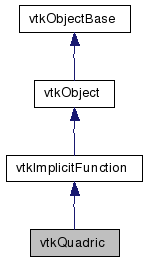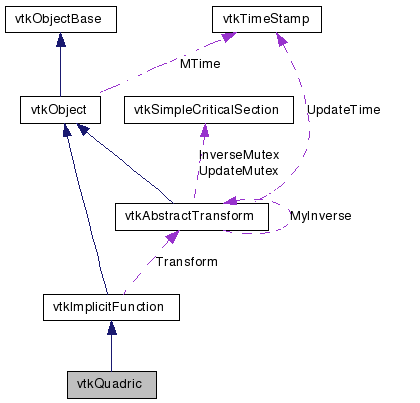`#include <vtkQuadric.h>`[legend][legend]

## Detailed Description

vtkQuadric evaluates the quadric function F(x,y,z) = a0*x^2 + a1*y^2 + a2*z^2 + a3*x*y + a4*y*z + a5*x*z + a6*x + a7*y + a8*z + a9. vtkQuadric is a concrete implementation of vtkImplicitFunction.

Tests:

Definition at line 31 of file vtkQuadric.h.

## Public Types

typedef vtkImplicitFunction Superclass

## Public Member Functions

virtual const char * GetClassName ()
virtual int IsA (const char *type)
void PrintSelf (ostream &os, vtkIndent indent)
void EvaluateGradient (double x, double g)
double EvaluateFunction (double x)
double EvaluateFunction (double x, double y, double z)
void SetCoefficients (double a)
void SetCoefficients (double a0, double a1, double a2, double a3, double a4, double a5, double a6, double a7, double a8, double a9)
virtual double * GetCoefficients ()
virtual void GetCoefficients (double data)

## Static Public Member Functions

static int IsTypeOf (const char *type)

## Protected Attributes

double Coefficients 

## Member Typedef Documentation

Reimplemented from vtkImplicitFunction.

Definition at line 34 of file vtkQuadric.h.

## Constructor & Destructor Documentation

 vtkQuadric::vtkQuadric ( ) ` [protected]`

 vtkQuadric::~vtkQuadric ( ) ` [inline, protected]`

Definition at line 60 of file vtkQuadric.h.

## Member Function Documentation

 virtual const char* vtkQuadric::GetClassName ( ) ` [virtual]`

Reimplemented from vtkImplicitFunction.

 static int vtkQuadric::IsTypeOf ( const char * name ) ` [static]`

Return 1 if this class type is the same type of (or a subclass of) the named class. Returns 0 otherwise. This method works in combination with vtkTypeRevisionMacro found in vtkSetGet.h.

Reimplemented from vtkImplicitFunction.

 virtual int vtkQuadric::IsA ( const char * name ) ` [virtual]`

Return 1 if this class is the same type of (or a subclass of) the named class. Returns 0 otherwise. This method works in combination with vtkTypeRevisionMacro found in vtkSetGet.h.

Reimplemented from vtkImplicitFunction.

 static vtkQuadric* vtkQuadric::SafeDownCast ( vtkObject * o ) ` [static]`

Reimplemented from vtkImplicitFunction.

 void vtkQuadric::PrintSelf ( ostream & os, vtkIndent indent ) ` [virtual]`

Methods invoked by print to print information about the object including superclasses. Typically not called by the user (use Print() instead) but used in the hierarchical print process to combine the output of several classes.

Reimplemented from vtkImplicitFunction.

 static vtkQuadric* vtkQuadric::New ( ) ` [static]`

Construct quadric with all coefficients = 1.

Reimplemented from vtkObject.

 double vtkQuadric::EvaluateFunction ( double x ) ` [virtual]`

Implements vtkImplicitFunction.

 double vtkQuadric::EvaluateFunction ( double x, double y, double z ) ` [inline]`

Reimplemented from vtkImplicitFunction.

Definition at line 43 of file vtkQuadric.h.

 void vtkQuadric::EvaluateGradient ( double x, double g ) ` [virtual]`

Implements vtkImplicitFunction.

 void vtkQuadric::SetCoefficients ( double a )

Set / get the 10 coefficients of the quadric equation.

 void vtkQuadric::SetCoefficients ( double a0, double a1, double a2, double a3, double a4, double a5, double a6, double a7, double a8, double a9 )

Set / get the 10 coefficients of the quadric equation.

 virtual double* vtkQuadric::GetCoefficients ( ) ` [virtual]`

Set / get the 10 coefficients of the quadric equation.

 virtual void vtkQuadric::GetCoefficients ( double data ) ` [virtual]`

Set / get the 10 coefficients of the quadric equation.

## Member Data Documentation

 double vtkQuadric::Coefficients` [protected]`

Definition at line 60 of file vtkQuadric.h.

The documentation for this class was generated from the following file:

Generated on Wed Jun 3 19:29:53 2009 for VTK by1.5.6